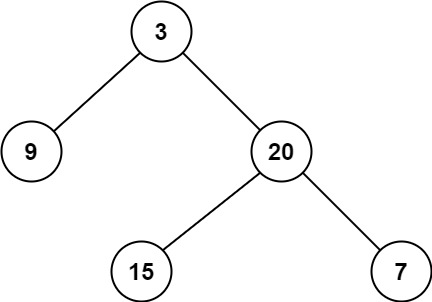# Algo.Leetcode.111. Minimum Depth of Binary Tree

Given a binary tree, find its minimum depth.

The minimum depth is the number of nodes along the shortest path from the root node down to the nearest leaf node.

Note: A leaf is a node with no children.

Example 1:```Input: root = [3,9,20,null,null,15,7]
Output: 2
```

Example 2:

```Input: root = [2,null,3,null,4,null,5,null,6]
Output: 5
```

Constraints:

• The number of nodes in the tree is in the range `[0, 105]`.
• `-1000 <= Node.val <= 1000`

Decision

``````/**
* Definition for a binary tree node.
* public class TreeNode {
*     public int val;
*     public TreeNode left;
*     public TreeNode right;
*     public TreeNode(int val=0, TreeNode left=null, TreeNode right=null) {
*         this.val = val;
*         this.left = left;
*         this.right = right;
*     }
* }
*/
public class Solution {
public int MinDepth(TreeNode root) {

if (root  == null)
return 0;

var left = 0;
var right = 0;

Console.WriteLine("root {0} left {1} right {2}", root.val, root.left, root.right);

if (root.left == null && root.right == null) {
return 1;
}

if (root.left != null) {
left =  1 + MinDepth(root.left);
}

if (root.right != null) {
right = 1 + MinDepth(root.right);
}

if (left != 0 && right != 0)
return Math.Min(left, right);
else
return Math.Max(left, right);
}
}``````
This entry was posted in Без рубрики. Bookmark the permalink.